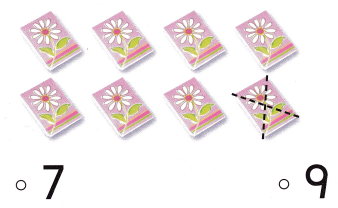# Texas Go Math Kindergarten Lesson 8.7 Answer Key One More and One Less

Refer to our Texas Go Math Kindergarten Answer Key Pdf to score good marks in the exams. Test yourself by practicing the problems from Texas Go Math Kindergarten Lesson 8.7 Answer Key One More and One Less.

## Texas Go Math Kindergarten Lesson 8.7 Answer Key One More and One Less

Explore

DIRECTIONS: Place 10 counters in the ten frame. Take one counter away. Write the number that is one less than 10. Place 10 new counters in the ten frame. Add one more counter. Write the number that is one more than 10.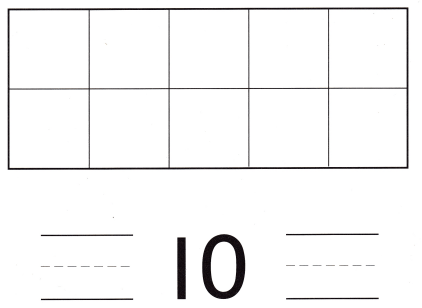Explanation:
One less than 10 = 10 – 1 = 9 or Nine.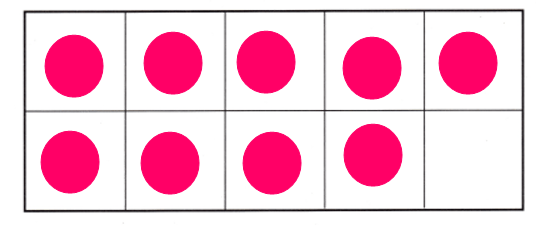One more than 10 = 10 + 1 = 11 or Eleven.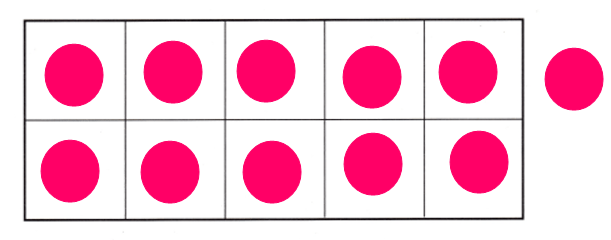Share and Show

DIRECTIONS: 1. There are 12 socks. Write the number that is one more than 12. Write the number that is one less than 12. 2. There are 15 hats. Write the number that is one more than 15. Write the number that is one less than 15.

Question 1.Explanation:
Number of socks = 12 or Twelve.
One more than 12 = 12 + 1 = 13 or Thirteen.

Question 2.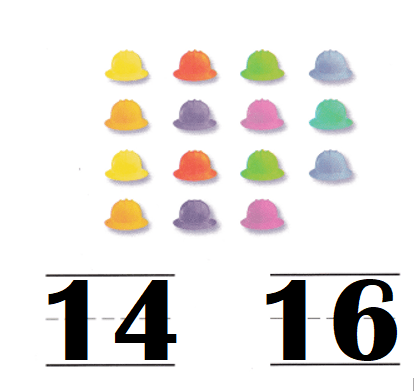Explanation:
Number of hats = 15 or Fifteen.
One more than 15 = 15 + 1 = 16 or Sixteen.
One less than 15 = 15 – 1 = 14 or Fourteen.

DIRECTIONS: 3-5. Look at the number. Write the number that is one less. Write the number that is one more.

Question 3.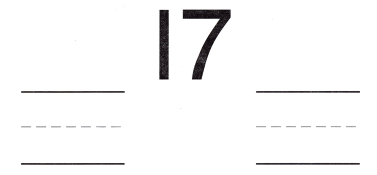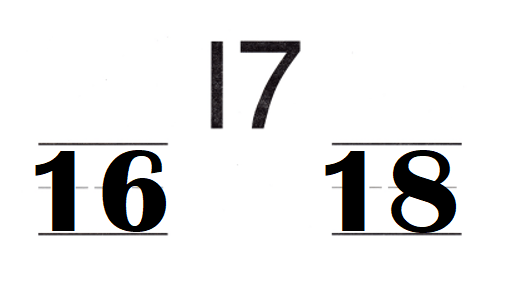Explanation:
One more than 17 = 17 + 1 = 18 or Eighteen.
One less than 17 = 17 – 1 = 16 or Sixteen.

Question 4.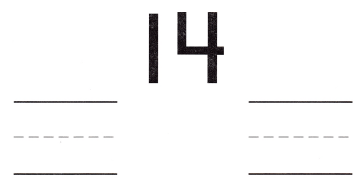Explanation:
One more than 14 = 14 + 1 = 15 or Fifteen.
One less than 14 = 14 – 1 = 13 or Thirteen.

Question 5.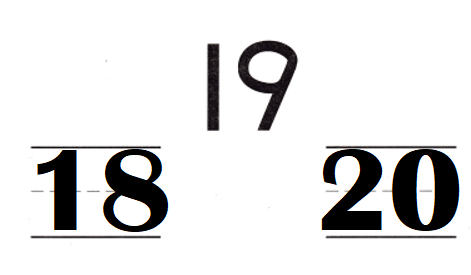Explanation:
One more than 19 = 19 + 1 = 20 or Twenty.
One less than 19 = 19 – 1 = 18 or Eighteen.

HOME ACTIVITY • Show your child a set of 11 to 20 objects such as spoons or forks. Have your child create a set of objects that shows one more or one less.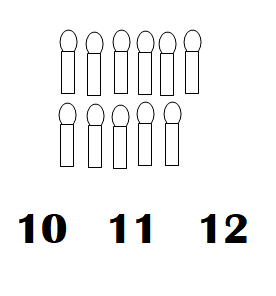Explanation:
My kid took spoons of 11.
One less than 11 = 11 – 1 = 10 or Ten.
One more than 11 = 11 + 1 = 12 or Twelve.

DIRECTIONS: 6. Adele has 10 hats. Seamus has one more hat than Adele. Draw to show how many hats Seamus has. 7. Choose the correct answer. There are 15 cubes. What number is one less?

Problem Solving
Question 6.Explanation:
Number of hats Adele has = 10.
Seamus has one more hat than Adele.
=> Number of hats Seamus has = Number of hats Adele has  + 1
= 10 + 1
= 11 or Eleven.

Question 7.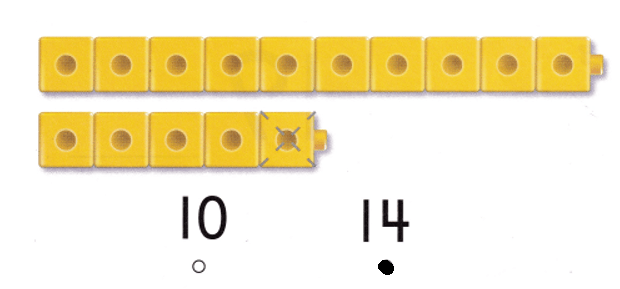Explanation:
Number of cubs = 15 or Fifteen.
One less than 15 = 15 – 1 = 14 or Fourteen.

### Texas Go Math Kindergarten Lesson 8.7 Homework and Practice Answer Key

DIRECTIONS: 1-3. Look at the number. Write the number that is one less. Write the number that is one more.

Question 1.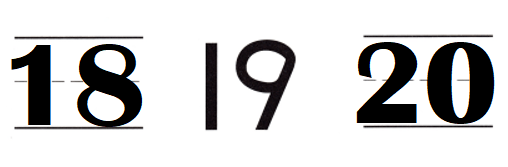Explanation:
One more than 19 = 19 + 1 = 20 or Twenty.
One less than 19 = 19 – 1 = 18 or Eighteen.

Question 2.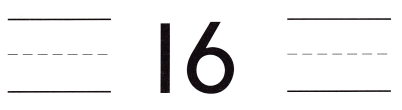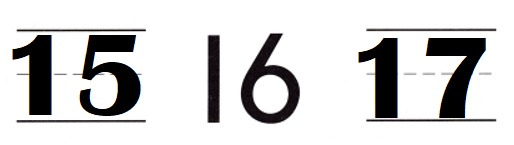Explanation:
One more than 16 = 16 + 1 = 17 or Seventeen.
One less than 16 = 16 – 1 = Fifteen.

Question 3.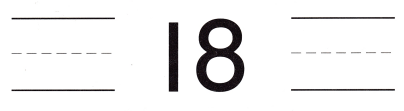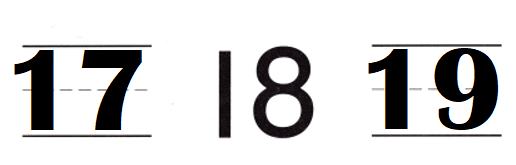Explanation:
One more than 18 = 18 + 1 = 19 or Nineteen.
One less than 18 = 18 – 1 = 17 or Seventeen.

DIRECTIONS: Choose the correct answer. 4. There are 6 cubes. What number is one less? 5. There are 11 stars. What number is one more? 6. There are 8 books. What number is one less?

Lesson Check
Question 4.Explanation:
Number of cubes = 16.
One less than 16 = 16 – 1 = 15 or Fifteen.

Question 5.Explanation:
One more than 11 stars = 11 + 1 = 12 or Twelve.

Question 6.